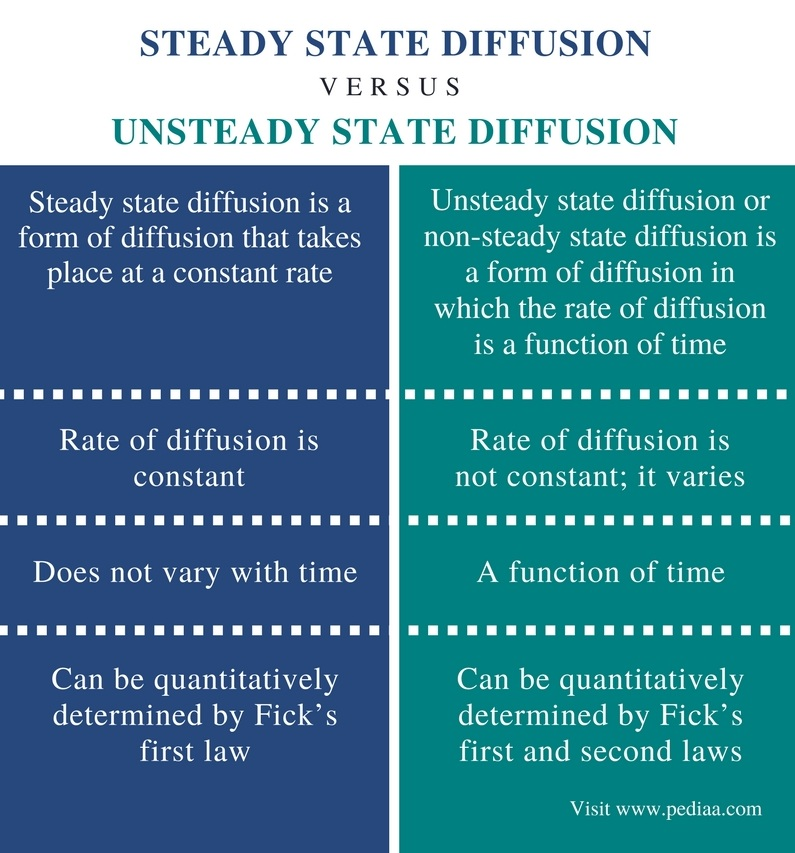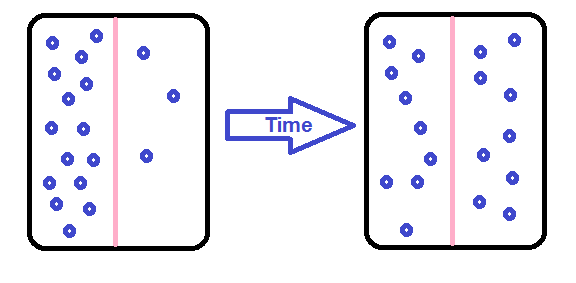At any temperature that is different from absolute zero, all atoms of a substance (in a gas, liquid or solid) are constantly in motion. These movements cause collisions with each other. Due to these collisions, the movement of particles seems zig-zag. However, it is observable that particles in a high concentration tend to move towards a lower concentration across a concentration gradient known as diffusion. Diffusion can be divided into two types as steady state diffusion and unsteady state diffusion. The main difference between steady state diffusion and unsteady state diffusion is that steady state diffusion takes place at a constant rate whereas the rate of unsteady state diffusion is a function of time. Both these types can be quantitatively described by Fick’s laws.

### Key Areas Covered

1. What is Steady State Diffusion
– Definition, Relationship with Fick’s First Law
2. What is Unsteady State Diffusion
– Definition, Relationship with Fick’s First and Second Laws
3. What is the Difference Between Steady State and Unsteady State Diffusion
– Comparison of Key Differences

Key Terms: Collisions, Diffusion, Fick’s Law, Steady State Diffusion, Unsteady State Diffusion## What is Steady State Diffusion

Steady state diffusion is a form of diffusion that takes place at a constant rate. Here, the number of moles of particles that cross a given interface is constant with time. Therefore, throughout the system, the rate of change of the concentration with distance (dc/dx) is a constant value and the change of concentration with time is zero (dc/dt).

dc/dx = constant

dc/dt = 0

Where dc is a change in concentration, dx is a small distance and dt is a small time period.Figure 1: Diffusion

Fick’s first law determines both steady state diffusion and unsteady state diffusion quantitatively. The Fick’s first law says that diffusive flux is directly proportional to the existing concentration gradient and it can be mathematically given as,

J   =   -D (dϕ/dx)

In which,

• J is the diffusion flux; its dimension is the amount of substance per unit area per unit time, and the unit is mol m−2s−1.
• D is the diffusion coefficient. It is also known as the diffusivity. The dimension of this component is area per unit time, hence the unit is m2/s.
• ϕ is the concentration. It is given by the unit mol/m3.
• x is the position of a solute. The dimension for this component is length. It is given by unit m.

## What is Unsteady State Diffusion

Unsteady state diffusion or non-steady state diffusion is a form of diffusion in which the rate of diffusion is a function of time. This means the rate of diffusion is time-dependent. Therefore, the rate of the concentration with distance (dc/dx) is not a constant and the change of concentration with time is not zero.

dc/dx = vary with time

dc/dt  ≠ 0

Where dc is a change in concentration, dx is a small distance and dt is a small time period.

Fick’s second law determines unsteady state diffusion quantitatively. Fick’s second law of diffusion is used to predict how the concentration changes with time when diffusion occurs. It is given by a partial differentiation equation as below.

δϕ/δt   = D  δ2ϕ/δx2

In which,

• ϕ is the concentration (a dimension that depends on time and the location (x)).
• t is time (given by s)
• D is the diffusion coefficient.
• X is the position (given by dimensions of length).

Hence, the unsteady state is formulated as a partial diffusion equation.

### Definition

Steady State Diffusion: Steady state diffusion is a form of diffusion that takes place at a constant rate.

Unsteady State Diffusion: Unsteady state diffusion or non-steady state diffusion is a form of diffusion in which the rate of diffusion is a function of time.

### Rate of Diffusion

Steady State Diffusion: The rate of diffusion is constant for steady state diffusion.

Unsteady State Diffusion: The rate of diffusion is not constant for unsteady state diffusion. It varies with time.

### Dependence on Time

Unsteady State Diffusion: Unsteady state diffusion is a function of time (it changes with time).

### Relationship to Fick’s laws

Steady State Diffusion: Steady state can be quantitatively determined by Fick’s first law.

Unsteady State Diffusion: Unsteady state diffusion can be quantitatively determined by Fick’s first and second laws.

### Conclusion

Steady state diffusion and unsteady state diffusion are two types of diffusion. Both these types can be quantitatively described by Fick’s laws. The main difference between steady state diffusion and unsteady state diffusion is that steady state diffusion takes place at a constant rate whereas the rate of unsteady state diffusion is a function of time.

##### Reference:

1. “Diffusion mechanisms .” Chapter 5. Diffusion, Available here.
2. “Viscoelastic (Non Fickian) Diffusion.” The Canadian Journal of Chemical Engineering, vol. 83, Dec. 2005, pp. 913–915., Available here.
3. “Multiphysics Cyclopedia.” COMSOL, Available here.